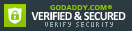ISBN: 0470099224

ISBN13: 9780470099223

# Solving Dynamics Problems in MATLAB to Accompany Engineering Mechanics Dynamics 6e

An introduction to MATLAB for engineering students, complete with practice problems Written as a complement to Engineering Mechanics Dynamics , this book provides students with an introduction to MATLAB as well as example problems that correspond to the aforementioned text. The book covers numerical calculations, defining functions, graphics, symbolic calculations, differentiation and integration, and solving equations with MATLAB and then presents...

## Selected

Format: Paperback

Condition: New

\$59.40

50 Available

## Customer Reviews

0 rating